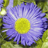cancel
Showing results for
Did you mean:

# PSoC™ 5, 3 & 1New Contributor II

## PSoC 1 analog subsections Information request

I'm currently a PhD ​Studentat National University of Technology, Argentina. I'm currently working on simulations of the analog sections of the CY8C29466-PXI PSoC, ​for evaluating the applicability of test methods. In order to improve the simulations accuracy I kindly ask, if you could provide me information about the following parameters (or a way to get them):

• The switches inside the SC Blocks and inside the capacitor arrays, particularly the on and off resistances
• Simulation models (SPICE) for the embedded Operational amplifiersor information that allows me to develop a behavioural-level simulation model.
• Analog muxes​, particularly the on and off resistances.
1 SolutionEmployee

## Re: PSoC 1 analog subsections Information request

Some of the information you want can be found in various device and component datasheets. For most users, the switch resistances and absolute capacitor values don't matter, because what matters is how well the circuit settles at the selected clock rate. But, as a guide, the unit capacitor value in the SCBlocks is 70 fF. I stated incorrectly in an earlier post (5 years ago) that the value was 50 fF. These are typical values, but to say it again, in most applications and most customers, it simply doesn't matter. I understand that you want to do simulations. So these numbers are important. Figure on SCBlock switch's resistance about 10kohm.

The opamp has gain of about 90 dB. The unity gain bandwidth is approximately 5.4 MHz at high power and high bias, each power reduction step cuts the GBW roughtly in half, so:

P=H,B=H, GBW = 5.4 MHz

P=H,B=L, GBW = 4 MHz (estimated)

P=M,B=H, GBW = 3.1 MHz

P=M,B=L, GBW = 2.5 MHz (estimated)

P=L,B=H, GBW = 015 MHz (estimated)

P=L, B=L, GBW = 0.75 MHz

Remember, the opamps can't go to the outside and must be buffered

The analog output buffers have a gain of 1.00 and a slew rate of 0.55 V/use into a 100 pF load.

Figure output resistance of buffer at 2 ohms.

You can construct a standard SPICE model from the DC gain and the GBW for both opamp and buffer.

The opamp bandwidth and slew rate get worse (lower) near Vss and Vdd,

In the SC blocks, switch resistance affects only settling time, not DC accuracy.

Mux on-resistance is equal to about 12 kohms. This is computed from stated mux capacitance and bandwidth.

This should get you started. If you have further questions, I am happy to help.

---- Dennis Seguine (longest serving PSoC applications engineer)

3 RepliesEmployee

## Re: PSoC 1 analog subsections Information request

Some of the information you want can be found in various device and component datasheets. For most users, the switch resistances and absolute capacitor values don't matter, because what matters is how well the circuit settles at the selected clock rate. But, as a guide, the unit capacitor value in the SCBlocks is 70 fF. I stated incorrectly in an earlier post (5 years ago) that the value was 50 fF. These are typical values, but to say it again, in most applications and most customers, it simply doesn't matter. I understand that you want to do simulations. So these numbers are important. Figure on SCBlock switch's resistance about 10kohm.

The opamp has gain of about 90 dB. The unity gain bandwidth is approximately 5.4 MHz at high power and high bias, each power reduction step cuts the GBW roughtly in half, so:

P=H,B=H, GBW = 5.4 MHz

P=H,B=L, GBW = 4 MHz (estimated)

P=M,B=H, GBW = 3.1 MHz

P=M,B=L, GBW = 2.5 MHz (estimated)

P=L,B=H, GBW = 015 MHz (estimated)

P=L, B=L, GBW = 0.75 MHz

Remember, the opamps can't go to the outside and must be buffered

The analog output buffers have a gain of 1.00 and a slew rate of 0.55 V/use into a 100 pF load.

Figure output resistance of buffer at 2 ohms.

You can construct a standard SPICE model from the DC gain and the GBW for both opamp and buffer.

The opamp bandwidth and slew rate get worse (lower) near Vss and Vdd,

In the SC blocks, switch resistance affects only settling time, not DC accuracy.

Mux on-resistance is equal to about 12 kohms. This is computed from stated mux capacitance and bandwidth.

This should get you started. If you have further questions, I am happy to help.

---- Dennis Seguine (longest serving PSoC applications engineer)New Contributor II

## Re: PSoC 1 analog subsections Information request

Thank you so much Dennis seg​. The information you've provided enables me to validate hypothesis and generate robust conclusions about them. As soon as the research results be come out, I'll be sharing them with the Developer Community.

----Emanuel DriEmployee

## Re: PSoC 1 analog subsections Information request